Run this tutorial

Click here to run this tutorial on mybinder.org:Please note that starting the notebook server may take a couple of minutes.

# Tutorial: Reducing an LTI system using balanced truncation¶

Here we briefly describe the balanced truncation method, for asymptotically stable LTI systems with an invertible $$E$$ matrix, and demonstrate it on the heat equation example from Tutorial: Linear time-invariant systems. First, we import necessary packages, including BTReductor.

import matplotlib.pyplot as plt
import numpy as np
import scipy.sparse as sps
from pymor.models.iosys import LTIModel
from pymor.reductors.bt import BTReductor

plt.rcParams['axes.grid'] = True


Then we build the matrices

import numpy as np
import scipy.sparse as sps

k = 50
n = 2 * k + 1

E = sps.eye(n, format='lil')
E[0, 0] = E[-1, -1] = 0.5
E = E.tocsc()

d0 = n * [-2 * (n - 1)**2]
d1 = (n - 1) * [(n - 1)**2]
A = sps.diags([d1, d0, d1], [-1, 0, 1], format='lil')
A[0, 0] = A[-1, -1] = -n * (n - 1)
A = A.tocsc()

B = np.zeros((n, 2))
B[:, 0] = 1
B[0, 0] = B[-1, 0] = 0.5
B[0, 1] = n - 1

C = np.zeros((3, n))
C[0, 0] = C[1, k] = C[2, -1] = 1


and form the full-order model.

fom = LTIModel.from_matrices(A, B, C, E=E)


## Balanced truncation¶

As the name suggests, the balanced truncation method consists of finding a balanced realization of the full-order LTI system and truncating it to obtain a reduced-order model.

The balancing part is based on the fact that a single LTI system has many realizations. For example, starting from a realization

\begin{split}\begin{align} E \dot{x}(t) & = A x(t) + B u(t), \\ y(t) & = C x(t) + D u(t), \end{align}\end{split}

another realization can be obtained by replacing $$x(t)$$ with $$T \tilde{x}(t)$$ or by pre-multiplying the differential equation with an invertible matrix. In particular, there exist invertible transformation matrices $$T, S \in \mathbb{R}^{n \times n}$$ such that the realization with $$\tilde{E} = S^{\operatorname{T}} E T = I$$, $$\tilde{A} = S^{\operatorname{T}} A T$$, $$\tilde{B} = S^{\operatorname{T}} B$$, $$\tilde{C} = C T$$ has Gramians $$\tilde{P}$$ and $$\tilde{Q}$$ satisfying $$\tilde{P} = \tilde{Q} = \Sigma = \operatorname{diag}(\sigma_i)$$, where $$\sigma_i$$ are the Hankel singular values (see Tutorial: Linear time-invariant systems for more details). Such a realization is called balanced.

The truncation part is based on the controllability and observability energies. The controllability energy $$E_c(x_0)$$ is the minimum energy (squared $$\mathcal{L}_2$$ norm of the input) necessary to steer the system from the zero state to $$x_0$$. The observability energy $$E_o(x_0)$$ is the energy of the output (squared $$\mathcal{L}_2$$ norm of the output) for a system starting at the state $$x_0$$ and with zero input. It can be shown for the balanced realization (and same for any other realization) that, if $$\tilde{P}$$ is invertible, then

$E_c(x_0) = x_0 \tilde{P}^{-1} x_0, \quad E_o(x_0) = x_0 \tilde{Q} x_0.$

Therefore, states corresponding to small Hankel singular values are more difficult to reach (they have a large controllability energy) and are difficult to observe (they produce a small observability energy). In this sense, it is then reasonable to truncate these states. This can be achieved by taking as basis matrices $$V, W \in \mathbb{R}^{n \times r}$$ the first $$r$$ columns of $$T$$ and $$S$$, possibly after orthonormalization, giving a reduced-order model

\begin{split}\begin{align} \hat{E} \dot{\hat{x}}(t) & = \hat{A} \hat{x}(t) + \hat{B} u(t), \\ \hat{y}(t) & = \hat{C} \hat{x}(t) + D u(t), \end{align}\end{split}

with $$\hat{E} = W^{\operatorname{T}} E V$$, $$\hat{A} = W^{\operatorname{T}} A V$$, $$\hat{B} = W^{\operatorname{T}} B$$, $$\hat{C} = C V$$.

It is known that the reduced-order model is asymptotically stable if $$\sigma_r > \sigma_{r + 1}$$. Furthermore, it satisfies the $$\mathcal{H}_\infty$$ error bound

$\lVert H - \hat{H} \rVert_{\mathcal{H}_\infty} \leqslant 2 \sum_{i = r + 1}^n \sigma_i.$

Note that any reduced-order model (not only from balanced truncation) satisfies the lower bound

$\lVert H - \hat{H} \rVert_{\mathcal{H}_\infty} \geqslant \sigma_{r + 1}.$

## Balanced truncation in pyMOR¶

To run balanced truncation in pyMOR, we first need the reductor object

bt = BTReductor(fom)


Calling its reduce method runs the balanced truncation algorithm. This reductor additionally has an error_bounds method which can compute the a priori $$\mathcal{H}_\infty$$ error bounds based on the Hankel singular values:

error_bounds = bt.error_bounds()
hsv = fom.hsv()
fig, ax = plt.subplots()
ax.semilogy(range(1, len(error_bounds) + 1), error_bounds, '.-')
ax.semilogy(range(1, len(hsv)), hsv[1:], '.-')
ax.set_xlabel('Reduced order')
_ = ax.set_title(r'Upper and lower $\mathcal{H}_\infty$ error bounds')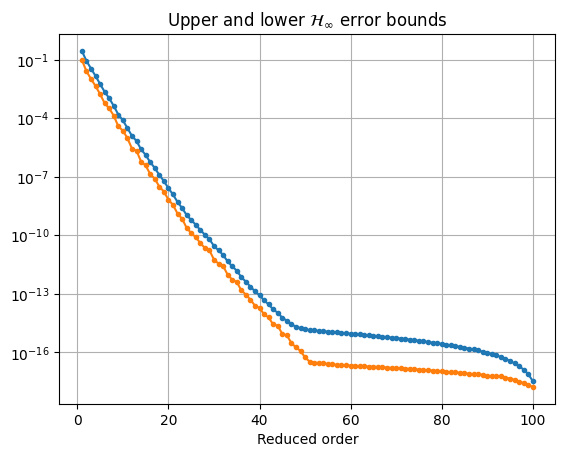To get a reduced-order model of order 10, we call the reduce method with the appropriate argument:

rom = bt.reduce(10)


Instead, or in addition, a tolerance for the $$\mathcal{H}_\infty$$ error can be specified, as well as the projection algorithm (by default, the balancing-free square root method is used). The used Petrov-Galerkin bases are stored in bt.V and bt.W.

We can compare the magnitude plots between the full-order and reduced-order models

w = np.logspace(-2, 8, 300)
fig, ax = plt.subplots()
fom.transfer_function.mag_plot(w, ax=ax, label='FOM')
rom.transfer_function.mag_plot(w, ax=ax, linestyle='--', label='ROM')
_ = ax.legend()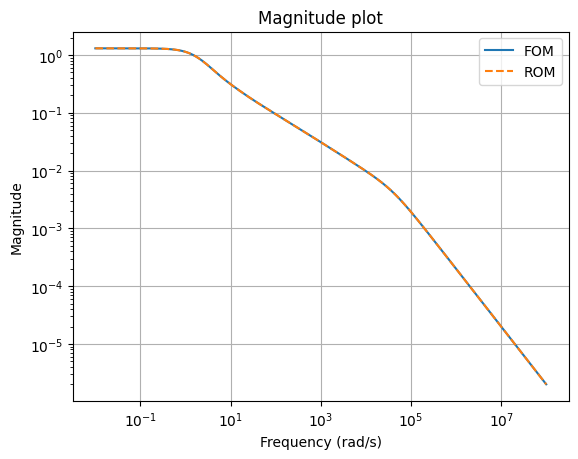as well as Bode plots

fig, axs = plt.subplots(6, 2, figsize=(12, 24), sharex=True, constrained_layout=True)
fom.transfer_function.bode_plot(w, ax=axs)
_ = rom.transfer_function.bode_plot(w, ax=axs, linestyle='--')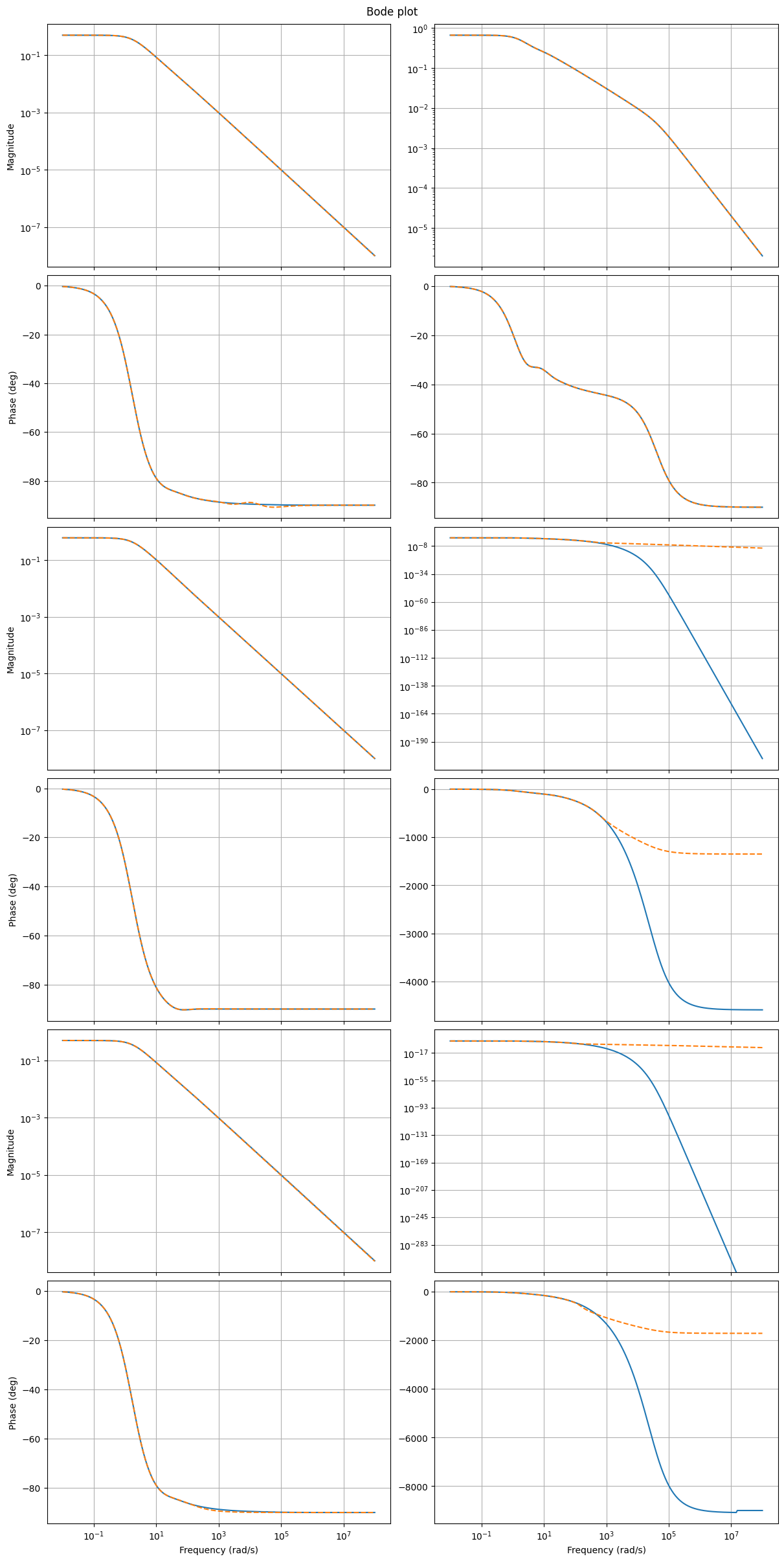Also, we can plot the magnitude plot of the error system, which is again an LTI system.

\begin{split}\begin{align} \begin{bmatrix} E & 0 \\ 0 & \hat{E} \end{bmatrix} \begin{bmatrix} \dot{x}(t) \\ \dot{\hat{x}}(t) \end{bmatrix} & = \begin{bmatrix} A & 0 \\ 0 & \hat{A} \end{bmatrix} \begin{bmatrix} x(t) \\ \hat{x}(t) \end{bmatrix} + \begin{bmatrix} B \\ \hat{B} \end{bmatrix} u(t), \\ y(t) - \hat{y}(t) & = \begin{bmatrix} C & -\hat{C} \end{bmatrix} \begin{bmatrix} x(t) \\ \hat{x}(t) \end{bmatrix}. \end{align}\end{split}
err = fom - rom
_ = err.transfer_function.mag_plot(w)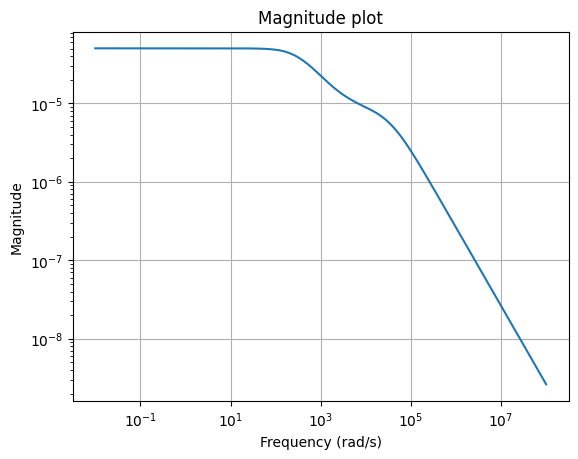and its Bode plot

_ = err.transfer_function.bode_plot(w)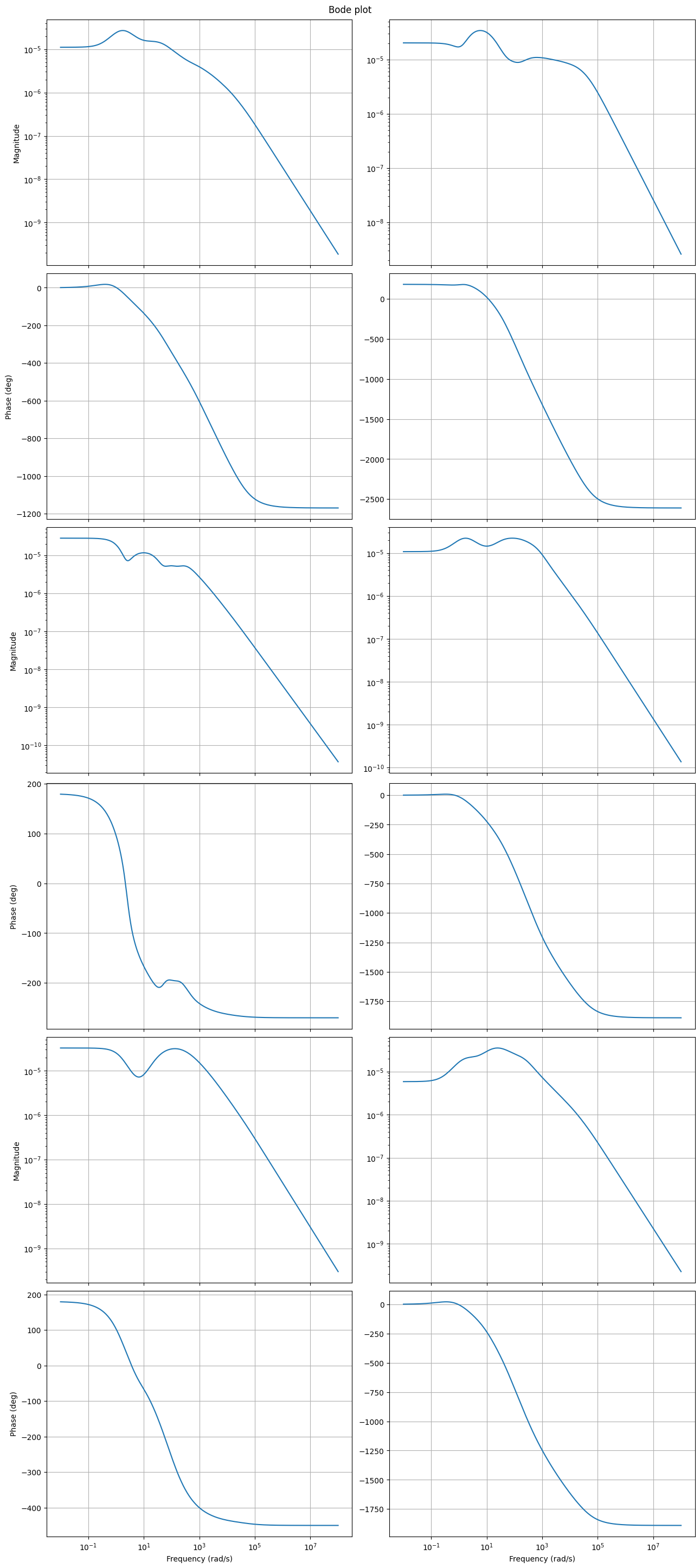Finally, we can compute the relative errors in different system norms.

print(f'Relative Hinf error:   {err.hinf_norm() / fom.hinf_norm():.3e}')
print(f'Relative H2 error:     {err.h2_norm() / fom.h2_norm():.3e}')
print(f'Relative Hankel error: {err.hankel_norm() / fom.hankel_norm():.3e}')

Relative Hinf error:   3.702e-05
Relative H2 error:     6.399e-04
Relative Hankel error: 7.629e-05


Download the code: tutorial_bt.md, tutorial_bt.ipynb.